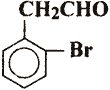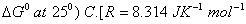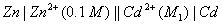Tuesday 29th September 2020

CBSE Guess > Papers > Question Papers > Class XII > 2003 > Chemistry > Compartment Delhi Set -I.

CHEMISTRY—2003 (Set I—Compartment Delhi)

Q. 1. Atomic number of sulphur is 16 and that of oxygen is 8. Calculate the total number of protons in a sulphite ion . 1

Q. 2. What is the possible value of angular momentum quantum number (1) for the unpaired electron in the atom of an element whose atomic number is 17? 1

Q. 3. In an alloy of gold and cadmium, gold crystallizes in cubic structure occupying the corners only and cadmium fits into the face centre voids. What is the quantitative composition of the alloy? 1

Q. 4. What will happen when red blood cells are placed in water? 1

Q. 5. Which solution will allow greater conductance of electricity, I M NaCI at 293 K or 1 M NaCl at 323 K? 1

Q. 6. Give one important industrial use of phenyl isocyanide. 1

Q. 7. Why is hydrogen sulphide, with greater molar mass, a gas, while water a liquid at room temperature?1

Q. 8. Mention two uses of pyrophoric alloys. 1

Q. 9. How does the addition of alum purify water? 1

Q. 10. What is 'codon'? 1

Q. 11. Write the de-Broglie education and establish a relation between wavelength of a moving subatomic particle and its kinetic energy. 2

Q. 12. An element occurs in BCC structure with cell edge of 300 pm. The density of the element is S. g cm -3 How many atoms of the element does 200 g of the element contain? 2

Q. 13. An aqueous solution of sodium chloride freezes below 273 K. Explain the lowering in freezing point of water with the help of a suitable diagram. 2

Q. 14. Calculate the volume of 80% (by mass) of H2 SO4 (density = 1.80 g/ml) required to prepare 1 litre of 0.2 molar H 2SO4 (Relative atomic masses: H = 1,O = 16, S = 32) 2

Q. 15. Evaporation of water is an endothermic process but spontaneous. Explain. 2

Q. 16. Explain the mechanism of chemical reaction between H 2 and Cl 2 in the presence of sunlight. Write any two important observations on such reactions.2

Q. 17. What will be the initial rate of reaction if its rate constant is 10 -3 s -1 and the concentration of the reactant is 0.2 mol L -1 ? What fraction of the reactant will be converted into the products in 200 seconds? 2

Q. 18. Account for the following:

(a) o-nitrophenol has lower boiling point than p-nitro-phenol.
(b) The dipole moment of chlorobenzene is less than that of methyl chloride.

Q. 19. Write the IUPAC names for the following: 2
(i)(ii) CH3 - CH2 - CH - COOH
|
Br

Q. 20. In contrast to arenes, aliphatic hydrocarbons do not undergo nitration. Explain. 2

Q. 21. Give chemical tests to distinguish between the following pairs of compounds:
(a) 1-nitropropane an 2-nitripropane 2
(b) CH 3 - CH 2 - NH 2 and (CH 3 ) 2 NH

Q. 22. How do the thermoplastic polymers differ from thermosetting polymers in their mode of formation? Give one example of each. 2

Q. 23. Why do lyophilic sols not require any stabilizing agent for their preservation? How is colloidal sulphur in water prepared? 2

Q. 24. What is 'genetic engineering'? Mention two of its main objectives. 2

Q. 25. Answer the following: 2
(a) How does respiration impart colour to blood?
(b) How do cells derive their need of ATP?

Q. 26. Give three examples of sulpha drugs and write their main uses. 2

Q. 27. (a) Illustrate with an example what is meant by standard enthalpy of formation of a compound.
(b) State the relation between standard free energy change and equilibrium constant of a chemical reaction.
0C for the processCalculate the value ofQ.28. The emf of the cellhas been found to be 0.305 V at 298 K. Calculate the value of M 1 .

Q. 29. How will you obtain the following:
(a) 1, 2etkaaedlol from ethanol
(b) 2-metIi from 2
(c) Benzoic acid from chlorbenzene
Give the complete chemical reaction and condition in each case.

Q. 30. What happens when (write reactions only): 3
(a) Methoxybenzene Is subjected to nitration.
(b) Ethanamide is reacted with HNO2.
(c) Acetic acid is reacted with chlorine in the presence of red phosphorus.

Q. 31. (a) A coordination compound has the formula CoCI 3 . 4NH 3 . It does not liberate ammonia but forms a precipitate with AgNO 3 . Write the structure and IUPAC name of the complex compound.
(b) Name a ligand. Which is bidentate and give an example of the complex formed by this ligand. 3

Q. 32. (a) State the principle of 'neutron activation analysis'.
(b) A sample of U-238 (half -life 4.5 x 109 years) ore is found to contain 23.8 g of U-238 and 20.6 g of Pb-206. Calculate the age of the ore. 3

Q. 33. Explain the following observations: 5
(a) Hydrogen fluoride has the highest boiling point among th hydrogen halides.
(b) Although I.E. of lithium is maximum amongst Group 1 metals, it is the Strongest reducing agent in solution.
(c) Sodium metal can be used for drying ether but cannot be used for drying
(d) A nitrogen atom has five valence electrons but it does not form the compound NCI 5 .
(e) Solubility of sulphates of Group 2 elements in water decreases down the group.

Q. 34. (a) Explain the following:
(i) The transition elements have high enthalpies of atomization.
(ii) The d-block elements exhibit more number of oxidation states than do the f-block elements.
(b) A green chromium compound 'A' on fusion with alkali gives a yellow compound 'B' which on acidification gives an orange coloured solution 'C'. 'C' on treatment with NH4 CI gives a coloured product 'D' which on crystallization and subsequent heating decomposes to give back compound 'A' identify A, B, C and D. Write equations for the reactions involved. 5

 Chemistry 2003 Question Papers Class XII Delhi Outside Delhi Compartment Delhi Compartment Outside DelhiSet ISet ISet ISet ISet IISet IISet IISet II

CBSE 2003 Question Papers Class XII